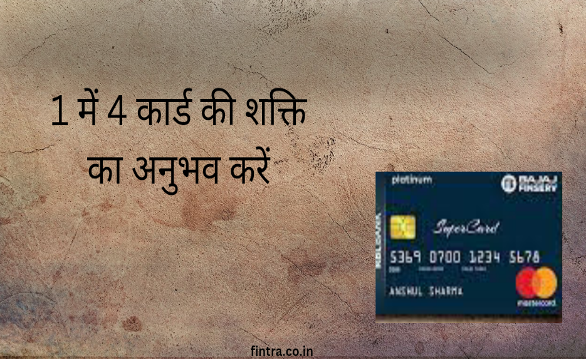-->

# Bike Loan EMI Calculator

Fintra’s easy to understand Bike Loan EMI Calculator helps you calculate the EMI required to pay for Bike Loan.

Try FintraGPT now.

## What is Bike Loan EMI?

EMI is a short form of “Equated Monthly Instalment”. Bike Loan EMI includes the repayment of principal as well as interest amount on your Bike loan. EMI primarily depends upon Amount, Rate of interest, and Time period. The longer the time period and lesser would be the EMI, but you will end up paying more interest to your lender

## What is Bike Loan EMI Calculator?

Fintra has come with a very effective tool so that you can calculate your Bike Loan EMI easily depending on various factors like rate of interest, tenure of the loan, etc. This will help you calculate the Bike loan.

## How to use Fintra's Bike Loan EMI Calculator?

All you need to do is input the following to arrive at your Bike Loan EMI:

Loan Amount: Enter the Loan Amount that you need for your Bike

Interest Rate (% per annum): Input prevailing interest rate that bank is charging

Loan Tenure (in years): Enter your desired loan term for which you wish to avail of the loan. A longer tenure increases the chances of getting loan approval.

## What are the benefits of using Fintra’s Bike Loan EMI calculator?

1. Helps you decide the EMI accurately without any errors and helps you make a desired plan that how you intend to pay back the EMI.

2. Saves your time which you would have wasted doing a long calculation to calculate the EMI amount which is a very exhaustive and time-consuming process.

3. You can access our EMI calculator anytime anywhere on our website or from our app.

How is Bike Loan EMI calculated?

The formula used to calculate the EMI is

EMI = [P x R x (1+R) ^N]/[(1+R) ^N-1]

Where P = Loan amount

R = Rate of interest

N = Tenure in number of months

Using the formula, you now have an idea that higher the loan amount or the rate of interest higher the EMI. Well the EMI payments decrease with the increase in tenure. But why get into this much hassle when you can do it all much more efficiently using an EMI calculator.

What are the factors that your Bike Loan EMI is dependent upon?

1. Amount borrowed – EMI is dependent on the amount and the interest is also calculated on this principal amount.
2. Rate of Interest- It is the rate at which interest will be computed. It is advisable to check the rate of interest of different banks before making a final choice.
3. Tenure of loan- It refers to the total amount of time in which the repayment will be done.
4. Monthly resting period – Resting period is the regular interval at which the loan amount balance is recalculated and refers to the periodicity of compounding. For example, in the case of a monthly rest, the balance loan amount is recalculated and decreases every month.

Other Factors affecting Bike Loan EMI

• If the borrower pays the loan by making a pre-payment through the tenure of the loan, either the subsequent EMI’s get reduced or the tenure is reduced or even both can happen. In the opposite case if the borrower skips an EMI then the EMI’s increase or the tenure increases or even both.

• Also, in case of floating rate loans if the rate of interest decreases the EMI reduces or the tenure decreases or even both. On an increase in rate of interest the reverse happens.

Flexible loan repayment option

Some banks offer flexible repayment of loans option in which the EMI’s vary over time. In step-up loans, you pay lower EMI in starting which eventually increases. In cases of step-down loans, you pay higher EMI in starting which decreases over the period.

### Recommended Blogs###### भारत में 5 शीर्ष बजाज फिनसर्व आरबीएल क्रेडिट कार्ड 2023###### कैसे वॉलेट केयर बजाज फिनसर्व आपके वॉलेट को ओल्ड स्थिति में रखने में मदद करता है###### एचडीएफसी मिलेनिया क्रेडिट कार्ड बनाम रेगलिया फर्स्ट क्रेडिट कार्ड###### How Wallet Care Bajaj Finserv Helps To Keep Your Wallet in Pristine Condition###### 5 Top Bajaj Finserv RBL Credit Cards in India 2023